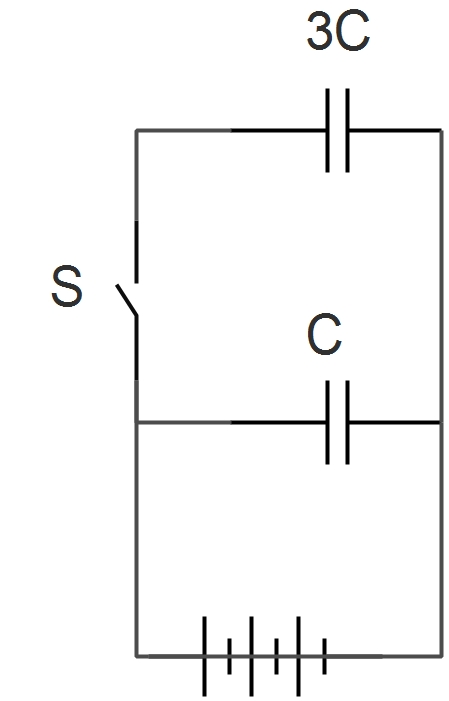# Two Different ThingsTwo identical parallel plate capacitors are connected to a battery with the switch $S$ closed. When $S$ is opened and the free space between the capacitors is filled with a material of dielectric constant $K$, the ratio of the total electrostatic energies stored in both capacitors, after and before the introduction of the dielectric, is $\text{\_\_\_\_\_\_\_\_\_\_}$.

×

Problem Loading...

Note Loading...

Set Loading...